## Kyle Thinks About Computer Vision

I think it is valuable to learn about the history of computer vision, especially when considering the ways in which it affects our everyday lives. CAPCHA has been used since the 90s to prevent computers from doing human things online such as open accounts and buy things. In the last couple years however, Computers have become so good at processing images, that these tests have become obsolete. As a result, CAPCHA has had to innovate and develop new methods of getting passed computers. (If you are curious about this, check out episode 908 of the podcast, Planet Money, titled “I Am Not A Robot.”Computer vision on its own is a powerful tool, and as the article discusses, can be used for many applications from  interactive artwork to measuring human reactions. When used in combination with machine learning however, a whole new world of applications opens up. It gives hackers new ways to grab information, and researchers have been able to use it to analyze the content of millions of  posts on social media. In this way, it is at the cutting edge of computer technology today.

But despite all the fancy applications, computer vision is just cool. There is something magical about being able to interact with a computer with only motion.

## Kyle plays with 3D pixels and may or may not have created an image of God

I worked with a friend to learn how to take an image that I had grabbed color values from and pixelated, and make each of the pixels into a 3-dimensional box. we used “brightness” to sense how bright each square was and then correlated that to the magnitude of the pixel’s height.

I also correlated the zoom and overall pixel height to mouse X and mouse Y via a map.

```PImage img;
float increment = 5;
float increment2 = 150;

void setup() {
size(700, 700, P3D);
imageMode(CENTER);
rectMode(CENTER);
}

void draw() {
noStroke();
background(0);
translate(50, 50);
rotateY(0);

// pushStyle();
// imageMode(CENTER);
// PImage section= img.get (mouseX,mouseY,50,50);
// image(section, mouseX,mouseY, 200, 200);
// //translate(50,50);

//popStyle();

for (int y = 0; y<img.height; y+= increment) {
for (int x = 0; x <img.width; x+= increment) {
int index = (x + y * img.width);
color pix = img.pixels[index];
fill(pix);
float diam = map(brightness(pix), 0, 255, 2, increment2/2);
pushMatrix();
translate(x, y, diam);
float a = map (mouseX, 0,700, 0, 20);
float b = map (mouseY, 0,700, 0, 20);
box(increment, increment, map(a, 0, b, 0, increment2));

popMatrix();

}
}

img.updatePixels();

}```

## Crossbow 2.0

With this weekly assignment, I attempt to create a Digital version of the analogue crossbow that I designed for my midterm, using the same 2 potentiometer, 1 button remote controller.

I spent quite a while fiddling with the code in the original handshake example, trying to get it to input data from multiple sensors connected to my Arduino. That being said, when I found the sketch that gave me the syntax to do it properly, I had most of the inputs properly formatted.

I ended up having to Add a third input to the code (for my button). Basically it would register pressed as a 1 and unpressed as a 0, then send it into the processing sketch, where I could make an if statement in order to draw a mark.

```void setup() {
Serial.begin(9600);
Serial.println("0,0,0");
pinMode(3, OUTPUT);
pinMode(5, OUTPUT);
pinMode(trigPin, INPUT);
}

void loop() {
while (Serial.available()) {
right = Serial.parseInt();
left = Serial.parseInt();

fire = 1;
}
else {
fire = 0;
}

digitalWrite(3, right);
digitalWrite(5, left);
delay(0);
delay(0);
Serial.print(sensor);
Serial.print(',');
Serial.print(sensor2);
Serial.print(',');
Serial.println(fire);

}
}

}```
```import processing.serial.*;
Serial myPort;
int xPos=0;
int yPos=0;
int button=0;
//boolean Button = false;
boolean onOff=false;
boolean onOff2=false;
//Array[] marks;
//Mark mark;

void setup() {
size(960, 720);
printArray(Serial.list());
String portname=Serial.list();
println(portname);
myPort = new Serial(this, portname, 9600);
myPort.clear();
myPort.bufferUntil('\n');
}

void draw() {
//background(255);
drawTarget();
drawCross();

if (button == 1) {
drawMark();
}

//if (keyPressed){
//  drawTarget();
//}

}

void serialEvent(Serial myPort) {
s=trim(s);
//t=trim(t);
if (s!=null) {
println(s);
int values[] = int (split(s, ','));
if (values.length ==3) {
xPos=(int)map(values, 0, 1023, 0, width);
yPos=(int)map(values, 0, 1023, 0, height);
button= (int) values;
}
}

myPort.write(int(onOff)+","+int(onOff2)+"\n");
}

void drawMark() {
noStroke();
fill(20, 15, 0);
ellipse (xPos,yPos, 10, 10);
}

void drawCross() {

fill(0, 255, 150);
noStroke();
rectMode(CENTER);
rect(xPos, yPos, 3, 30);
rect(xPos, yPos, 30, 3);
rect(xPos-20, yPos, 3, 15);
rect(xPos+20, yPos, 3, 15);
rect(xPos, yPos-20, 15, 3);
rect(xPos, yPos+20, 15, 3);
}

void drawTarget() {
fill(200, 118, 70, 240);
noStroke();
rectMode(CENTER);
rect(width/2, height/2, width, height);
fill(240);
rect(width/2, height/2, 400, 600);
fill(255, 255, 0, 150);
rect(width/2, height/2, 300, 450);
fill(240);
rect(width/2, height/2, 200, 300);
fill(255, 255, 0, 150);
rect(width/2, height/2, 100, 150);

fill(240);
rect(width*.17, height*.7, 150, 225);
fill(255, 255, 0, 150);
rect(width*.17, height*.7, 100, 150);
fill(240);
rect(width*.17, height*.7, 50, 75);

fill(240);
rect(width*.83, height*.7, 150, 225);
fill(255, 255, 0, 150);
rect(width*.83, height*.7, 100, 150);
fill(240);
rect(width*.83, height*.7, 50, 75);

fill(240);
rect(width*.17, height*.3, 150, 225);
fill(255, 255, 0, 150);
rect(width*.17, height*.3, 100, 150);
fill(240);
rect(width*.17, height*.3, 50, 75);

fill(240);
rect(width*.83, height*.3, 150, 225);
fill(255, 255, 0, 150);
rect(width*.83, height*.3, 100, 150);
fill(240);
rect(width*.83, height*.3, 50, 75);
}```

At the end, I attempted to make an array list in order to save points on the target that I had shot, but could not figure out how to make it work with my code.

## Can I “Command /” my Brain?: My relationship to Physical Computing

I keep finding myself wanting to press “command /” to comment out a part of an essay, or some thought I had, to save it for later. I have spent so much of my life actively avoiding learning how to program, it has been an interesting experience, finally getting into it. When working on a project, it feels like there is always some part of my mind trying to process what is happening in the code I am working, even when I am doing something else. In this way it has really become an interesting part of my life, that I never thought it would.

I feel Like I have improved my coding skills significantly, but with every weekly project, I feel like I end up kicking my own ass. Just one little conceptual misunderstanding can throw me off and send me into a downward spiral of confusion for hours. It is like trying to fly a plane without knowing what all the buttons do.

In that way, I really hate how limited i feel. It was much easier for me to conceptually understand physical things at the beginning of the semester, like circuitry and mechanical systems. Sometimes when I rely on just programming, I feel like I am sliding backwards down a slide, and end up feeling stupider than the day before.

I really want to keep improving my skills, but it is hard on my conscience and confidence to always be working just above the range of my ability.

Still, I am fascinated by computing. I wouldn’t keep working so hard at leaning it if I wasn’t. It helps me to see the world a little differently. I am really grateful that I stepped out of my comfort zone.

## The trees of the world: Data Visualization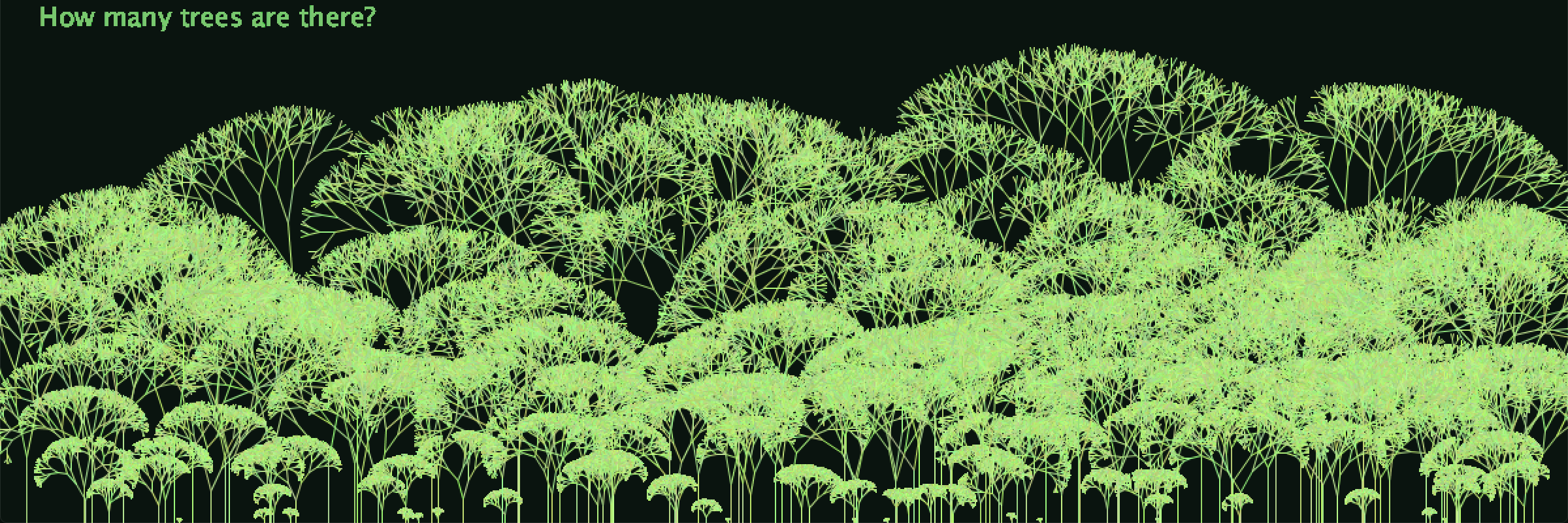This is how many trees there are(or at least how many there were in 2016).

I decided to do a data visualization of the percentage of land area covered by forest per country. The taller the tree, the bigger the percentage.

First I created a branch object, which I made into an array in my tree function, which I then make an array of in my draw function.

```ArrayList<Tree> trees;

float count = 0;
int data[];
Tree tree;
void setup() {
// I want to do three things,
//one create one tree for each point of data,
//two, make each tree's height dependent on the value of the data point
//three, be able to switch between data sets with a keypress. (less important)
size(1200, 400);
trees = new ArrayList<Tree>();
//if (mousePressed){
//}
//else {
//}
int[] data = new int[forest.length];

for(int i = 0; i < forest.length; i++){
data[i] = int(forest[i]);
}

for (int i = 0; i < data.length; i++) {
int x = int(random(20, width-20));
int y = int(random(height+4,height-4));
tree = new Tree(data[i], x, height );//enter height and position of tree
}
//float trunk = 50;
//float trunk = data.length;
}

void draw() {
background(10, 20, 15);
for (Tree tree : trees) {
tree.update();
tree.show();
fill(120,200, 110);
textSize(20);
text("How many trees are there?", 30, 20);

}
}```
```class Tree {
ArrayList<Branch> branches;
int trunk, x, y, count;

Tree(int trunk, int x, int y) {
branches = new ArrayList<Branch>();
this.trunk = trunk;
this.x = x;
this.y = y;
this.count = 0;

PVector a =  new PVector (x, y);
PVector b = new PVector (x, y-trunk);
Branch root = new Branch(a, b);
}

void update() {
if (count < 9) {
for (int i = branches.size() -1; i >= 0; i--) {
Branch current = branches.get(i);
//if the current Branch has no children: add them
if (!current.finished) {
}
//now that Branch has children
current.finished = true;
}
count ++;
}
}

void show() {
for (int i = 0; i < branches.size(); i++) {
branches.get(i).update();
}
}
}
```
```class Branch{

PVector start;
PVector end;
boolean finished = false;

Branch(PVector start,PVector  end){

this.start = start;
this.end = end;
}

void update() {

stroke(random(150,200),random(200,255),random(100,150));
line(start.x, start.y, end.x, end.y);

}

Branch branchA(){
PVector direction = PVector.sub(end, start);
direction.rotate(PI / random(4,20));
direction.mult(0.75);
Branch b = new Branch(end, newEnd);
return b;
}

Branch branchB(){
PVector dir = PVector.sub(end, start);
dir.rotate(- PI / random(4,20));
dir.mult(0.75);
Branch b = new Branch(end, newEnd);
return b;

}
}```

## Response to Digitize Everything: The Internet Lives

The main theme of this chapter of the book The Digitization of Just About Everything by Erik Brynjolfsson and Andrew McAfee (which I would like to note is a digital book, with PDF copies on sale for 8.95) is that the easy replication and distribution of digital information is the backbone of modern information systems such as the internet. Building off of this, they comment on how this creates a system in which it is difficult to produce information and digital products, but easy to reproduce them (there is nothing quite as useful in life as the copy paste function). I think their argument applies heavily to the work we do in class in multiple ways. For one, it applies literally. It takes time and energy to write code, but using functions such as classes, we are able to duplicate information that we have designed and spent time processing.

In a more metaphorical sense, however, This theory applies to students as well. There is a lot of effort needed to learn how to code. Once we have learned how to code however, we are able to more easily replicate, and even alter based upon what we have learned. Each of these systems evolves and grows over time as new information is added.

Another thing that the chapter details is how the immense amount of data on the internet (already measured in terms of zettabytes even in 2015 when it was written) serves to continuously allow the second machine age to progress, as more information equals the ability to further develop technology. As databases from academic search engines to wikipedia and even services like yelp expand, they become more accurate and expansive. Information is available at the press of a button, and it keeps getting better.

I’ve noticed it myself over the years. I was born in 1999, and was just barely able to understand the significance of the smartphone when the first Iphone came out in 2007. Since then, the effects of  Moores law have continuously unveiled  before my eyes. Sometimes it feels like I am growing at the same time as the internet. Sometimes it is difficult to distinguish the internet from a living, thinking thing. This is partly because of the similarity between how humans build information and experiences over the course of their lives, and how this web of interconnected information gathering processes does the same over the course of its life.

## SHAME GAME

```//Box B[];
//startScreen S;
RotateBox Box;
EvilSquare evil, evil1;
boolean overButton = false;
boolean buttonPress = false;
int qwerty = 100;

void setup() {
size(820, 640);
//  int lineLength = 30;
//  int w = width/lineLength;
//  int h = height/lineLength;
//B = new Box[w*h];
//float i = 0;
//S = new startScreen();
evil1 = new EvilSquare(qwerty);
evil = new EvilSquare(qwerty+100);
Box = new RotateBox();
}
void draw() {
background(175, 200, 250);
landscape();
Box.run();
startScrn();
if (buttonPress == true ){
//if (millis()% 10 == 0){
//translate(0,random(-300, 300));
evil.evilRun();
evil1.evilRun();
//for int i ==
textSize(25);
text("DEFEND!", width/2-50,height/2-10);

}}

void landscape() {
noStroke();
fill(20, 200, 125);
rect(0, height*.8, width, height);
noStroke();
fill(175, 180, 125);
triangle(width*.1-10, height*.75, width*.1+10, height*.75, width*.1, height*.75-100);
noStroke();
fill(20, 200, 125);
ellipse(width*.1, height*.8, 100, 100);
}

void checkButtons() {
if (mouseX > width*.4 && mouseX < width*.6 && mouseY > height*.4 && mouseY <height*.55) {
overButton = true;
}
}

void startScrn(){

if (buttonPress ==true){
noFill(); }
else {
stroke(random(255),random(255),random(255));
strokeWeight(5);
fill(230);
rect(width/20,height/20, width-width/10,height-height/10);
stroke(0);
strokeWeight(5);
fill(255);
rect(width*.4,height*.4, width*.2,height*.15);
fill(0);
text("start game", width*.46,height*.47);
}

}

//void reset(){

//  noFill();
//  stroke(0);
//  strokeWeight(3);
//  rect (width*.87,height*.9, 80, 30);
//  text("reset", width*.92,height*.92);
//}

void mousePressed() {
if (overButton && mousePressed) {
buttonPress = true ;}

}

void mouseMoved() {
checkButtons();
}

```

Sorry.

```class RotateBox {
PVector origin;
float angle;
int len;

RotateBox(){

origin = new PVector(width*.1, height*.75-100);
angle=0;
len =50;
}

void update() {

PVector mouse = new PVector(mouseX, mouseY);
PVector direction = PVector.sub(mouse, origin);//I want to subtract second from first one

}

void display() {

pushMatrix();
translate(width*.1, height*.75-100);
rotate(angle);

stroke(0);
strokeWeight(4);
fill(200,200,175);
rect(0, 0, len, 15);

popMatrix();
}
void run() {
update();
display();
}
}
```
```class EvilSquare {
float x;
float y;
int qwe;
EvilSquare(int _qwe){
qwe = _qwe;
x = width +100+qwe;
y = height/2;
}

void scare(){
x --;
}

void display() {

pushMatrix();

stroke(0);
fill(255,10, 10);
strokeWeight(0);
rect(x,y, random(20, 50), random (20, 50));

popMatrix();
}

void evilRun(){
scare();
display();
}

}```

## Designing for Outlires

In general, the human built environment is designed to fit the average person. Doors are designed for most people to be easily walked through. Chairs are designed to be sat in comfortable way (most of the time).As such, most things are designed to be functional to the greatest number of users: ie. Right handed scissors. As the article states, however, some of the most interesting functional design comes in to being as a result of variance from the norm.

The article explains the significance and design of a multitude of common, and less common objects, and how they were designed to equalize those with disabilities such as bad eyesight, hearing, and lack of limbs. I was fascinated by the way this article defined an object as common as eyeglasses through this lens.

So if you are a particularly tall person, design a door you can fit through.## Copying the first on screen computer art in code

For my project, I decided to copy Ben F. Laposky’s  sine wave Oscillons. Laposky created his original artwork starting in 1952, using oscillators, amplifiers, and modulators with a cathode ray tube to create what is generally regarded as the first computer art.

Since the image (presented here in both black and white and original) was created by taking pictures of analogue lines on a screen, I decided to simulate the motion of the original screen.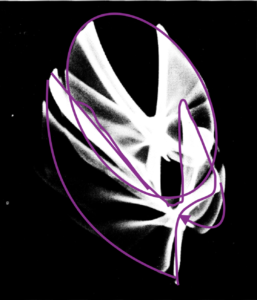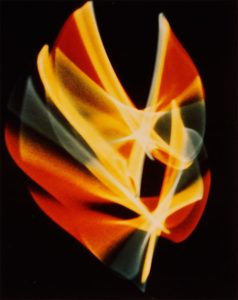```int t, x, y, x2, y2;
int a, b, b2, c, c2;
int d, e, e2, f, f2;
int w = 200;
float speed = .03;
float speed2 = .01;
float granulation = .0005;

void setup(){
size (480,640);
t = 0;
}

void draw(){

float frequency = (frameCount*speed) + (granulation);
float fillColor = map(noise(frequency), .01, .75, 0, 255);
float fillColor2 = map(sin(frequency), .01, .75, 0, 255);
float fillColor3 = map(cos(sin(frequency)), .01, .75, 0, 255);
fill(0,0,0,11);
rectMode(CORNER);
rectMode(CENTER);

//fill(100, 200, 3, fillColor);
stroke(fillColor, fillColor2, 3,fillColor);
strokeWeight(12);

pushMatrix();

float  t= frameCount*speed;

float x = radius * sin(t);
float y = radius * cos(t/8);

float x2 = radius/2 * sin(t-3);
float y2 = radius * cos(t);
translate(width/2,height/2);

line(x,y,x2,y2);

//popMatrix();
stroke(fillColor2, fillColor, fillColor,fillColor);
strokeWeight(12);
//pushMatrix();

float  a= frameCount*1.1*speed;

float c = radius * sin(a);
float b = radius * cos(a/2);

float c2 = radius * sin(a*1.3);
float b2 = radius * cos(a/3);
//translate(width/2,height/2);

line(c,b,c2,b2);

stroke(fillColor3, fillColor3, fillColor2,fillColor);
strokeWeight(12);
//pushMatrix();

float  d= frameCount*1.1*speed2;

float e = radius * sin(d);
float f = 20+ radius * cos(d/1.8);

float e2 = radius * sin(d/3);
float f2 = radius * cos(d/3);
//translate(width/2,height/2);

line(e,f,e2,f2);

//  float c = radius * sin(a(tan(a));
//float b = radius * cos(a/2);

//float c2 = radius * sin(a-3)*tan(a);
//float b2 = radius * cos(a/3);
////translate(width/2,height/2);

//  line(c,b,c2,b2);

popMatrix();

}```

To simulate the machine used to create the images, I decided to use bars or lines, making them mobile by having their endpoints following certain sin and cosine functions. I used a Trick Aaron taught me to blur it. The other main function of my code makes each bar change color within a certain specified range, either using noise or a sin/cos wave to change the values.

Once I figured this out, Most of what I had to do was play with where each bar was, what color it was, the speed, and blur.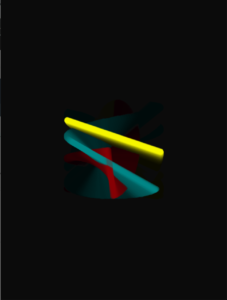```int t, x, y, x2, y2;
int a, b, b2, c, c2;
int d, e, e2, f, f2;
int w = 200;
float speed = .03;
float speed2 = .01;
float granulation = .0005;

void setup(){
size (480,560);
t = 0;
}

void draw(){

float frequency = (frameCount*speed) + (granulation);
float fillColor = map(noise(frequency*2), .01, .75, 190, 255);
float fillColor2 = map(sin(frequency), .01, .75, 190, 220);
float fillColor3 = map(cos(sin(frequency)), .01, .75, 0, 255);
fill(0,0,0,11);
rectMode(CORNER);
rectMode(CENTER);

//fill(100, 200, 3, fillColor);
stroke(fillColor, fillColor2, random(20),fillColor);
//stroke(200, 200, 3,fillColor);//test colors
strokeWeight(12);

pushMatrix();

float  t= frameCount*speed;

float x = (radius * -cos(t))+100;
float y = (radius * sin(t))+100;

float x2 = radius * cos(t);
float y2 = radius * -sin(t);
translate(width/2+20,height/2+-100);

line(x,y,x2,y2);

//popMatrix();
stroke(fillColor, fillColor2, 3,fillColor);
strokeWeight(12);
//pushMatrix();

float  a= frameCount*speed;

float c = (radius * -sin(a))+100;
float b = (radius * cos(a))+100;

float c2 = radius * sin(a);
float b2 = radius * -cos(a);
//translate(width/2+20,height/2-100);

line(c,b,c2,b2);

//          stroke(fillColor3, fillColor3, fillColor2,fillColor);
//        strokeWeight(12);
//          //pushMatrix();

//        float  d= frameCount*1.1*speed2;

//        float e = radius * sin(d);
//        float f = 20+ radius * cos(d/1.8);

//        float e2 = radius * sin(d/3);
//        float f2 = radius * cos(d/3);
//        //translate(width/2,height/2);

//          line(e,f,e2,f2);

//  float c = radius * sin(a(tan(a));
//float b = radius * cos(a/2);

//float c2 = radius * sin(a-3)*tan(a);
//float b2 = radius * cos(a/3);
////translate(width/2,height/2);

//  line(c,b,c2,b2);

popMatrix();

}```

Finally it was time for me to begin to copy the original image.

Here in this code, I have two systems working to create the first butterfly in the image. The color fluctuates within the spectrum from red/orange to yellow/green. What I need now is a second system, and possibly a way to translate it up and down as time goes in order to get the same quality as the original.

## Random and Organic Digital Art with Casey Reas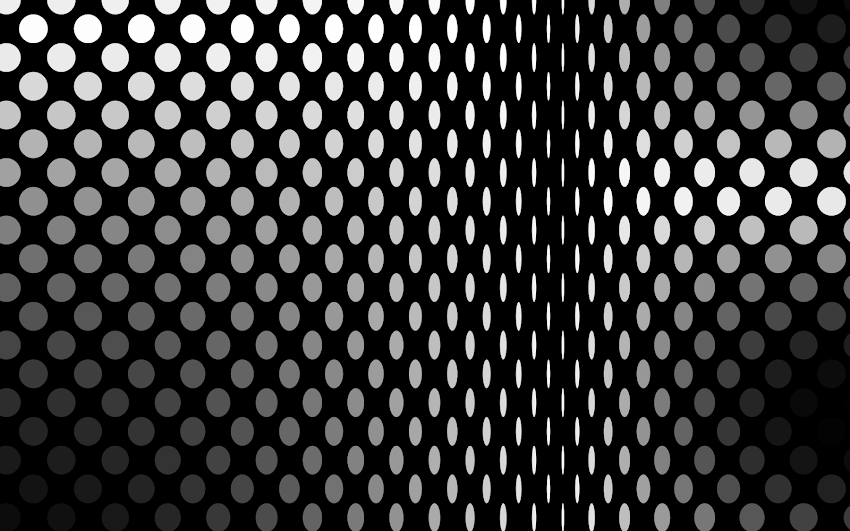What I found most fascinating about Casey Reas’ talk on his the theories of organic randomness and in digital artwork, is the way that he distilled the lines of code he writes into simple and direct paragraphs that define what Is going on. He then is able to combine those paragraphs, just like someone would while building interlaced code, and create a much more complex layering of Ideas and functions into a single image.

There is also a section where he discusses his desire to create a consistent moving texture that doesn’t decay after being played over the course of several days. This is interesting in context of one of Reas’ goals, to artificially create images that imitate organic life.

Another thing I was intrigued by was the program Reas used to demonstrate how symmetry can make something random into something recognizable, but organic.

I think it is interesting how he conceptualizes how the randomness of computer generated artwork has developed over time, and how he and others use it. He talks about it like any art critic would a piece of fine renaissance artwork, because this is the renaissance he sees. The digital age has opened up new fields and possibilities for the artwork we design.